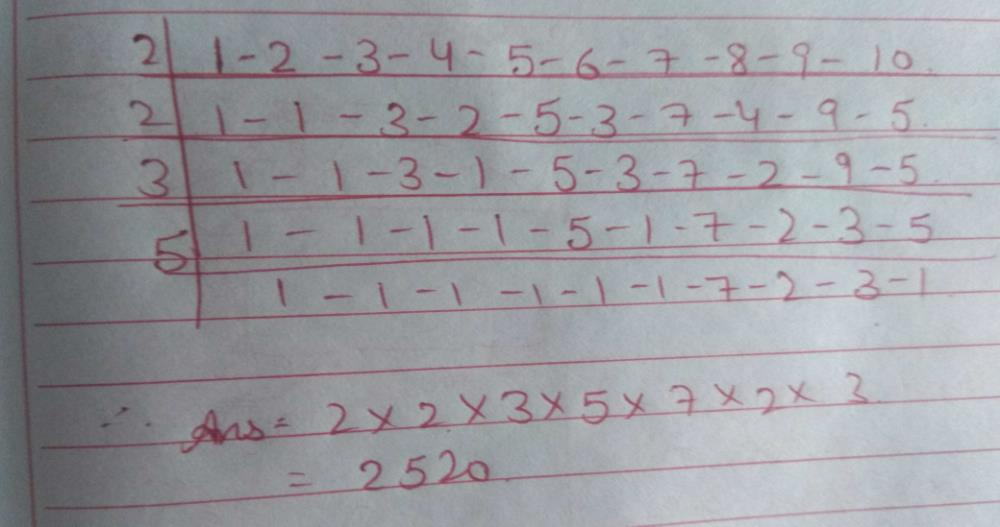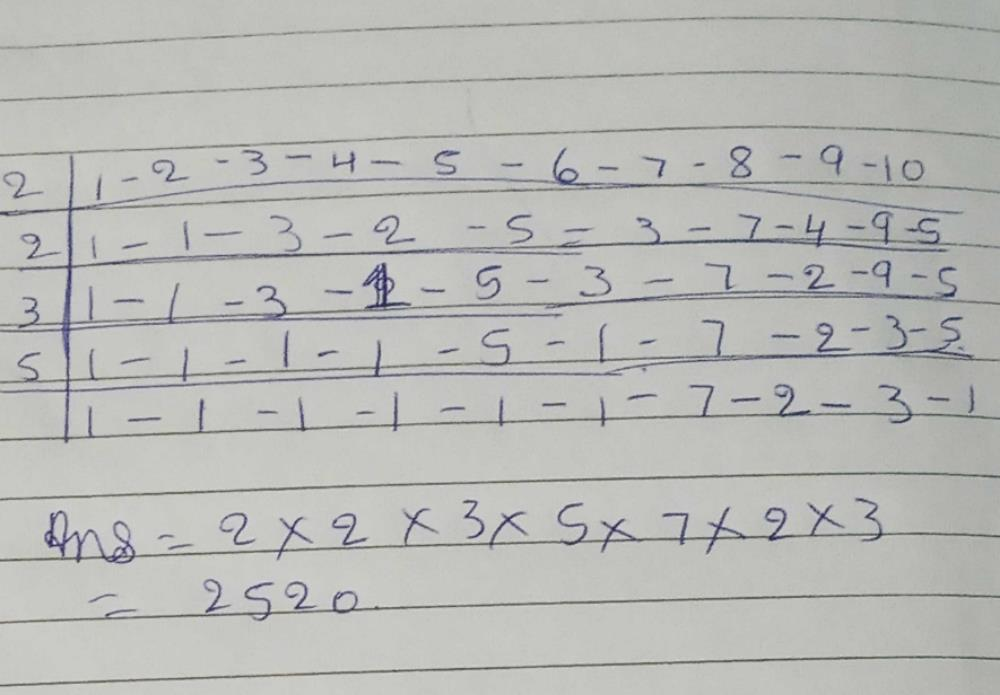Courses

# The least number that is divisible by all the numbers from 1 to 10 (both inclusive) isa)10b)100c)504d)2520Correct answer is option 'D'. Can you explain this answer? Related Test: Test: Knowing Our Number - 1

## Class 6 Question

By Sahil Gautam · Feb 08, 2020 ·Class 6
Monika Nehra answered Nov 11, 2019
2520Anshuman Kumar answered Jun 14, 2020

Ritu Ritu answered Feb 08, 2020
In this question why we take LCM

Vijender Chahal answered Apr 24, 2020
Correct answer is is 2520 because this number is divided by all the numbers came between 1 to 10.

D

Ravinder Gurwan answered Apr 11, 2020
Why

Chetan Rian answered Jun 24, 2020
The correct amswer is 2520

Sankalpa Sankalpa answered Apr 28, 2020
I think it's 10

Shashikant Gajakosh answered Jun 29, 2020Priyanka Biswas answered Jun 01, 2020

Jesus Ghosh answered May 11, 2020

100

Affan Modak answered Aug 26, 2020
(d) Factors of 1 to 10 numbersCool Clasher answered Apr 08, 2020

Biswanath Das answered Apr 07, 2020
1 to 10 divisible = 2520
=2×2×2×3×3×5×7
so,2520 will divisible by 1 to 10 .

Nukku Videos answered May 30, 2020
2520 is the write because it is divisible by all the numbers from 1 to 10

Barlin Mohamed answered May 10, 2020
2520 la the answer because we use LCM

Prema Latha answered Jul 03, 2020
I thinks 10 is the answer

Kavya Patel answered Jun 23, 2020

Anshu Ishwar answered Apr 16, 2020
(D)

Suryakanta Singh answered Apr 17, 2020
2520 is correct answer because all numbers from1-10
you know that 1 is divisible by any number and 2 is divisible by those numbers in which any even numbers came in last and odd number can divisible by those number which is in addition came odd like 2+5+2+0=9and odd numbers are divisible by 9 like 3,9.

Kothur Veeranna answered May 02, 2020
I did LCM and LCM with -1 - 1 - I did like this dancer came the answer came 2520 I am thinking I explains explains like in a picture in the phone I will submit it tomorrow

Anil Kumar answered Apr 19, 2020
2520 answer humera Lcm Lana Se aya

Shravani Sakure answered Jul 21, 2020
D)2520

Akshita Jaswal answered Aug 24, 2020
2520 is: the smallest number divisible by all integers from 1 to 10, i.e., it is their least common multiple. half of 7! (5040), meaning 7 factorial, or 1×2×3×4×5×6×7.

Falak Rajput answered May 09, 2020
As if we take the lcm then we would find that it is divisible by al no. from1 to 10

Anjanatanu Tanu answered Aug 15, 2020
Divisibility rules of numbers from 1 to 10
1- all numbers are divisibile
2-if last digit is even then divisibile
3- sum of the digits is multiple of 3 then divisibile
4-last two digits must be divisibile by 4
5- last digit must be 0 or 5
6- the digit must be divisibile by both 2 and 3
7-the last digit should be doubled and substracted by rest of the digit and the answer must be divisibile by 7
8-last 3 digits of the number should be divisibile by 8
9 -sum of number should be multiple of 9
10 - last digit must be zero

a) 10 is not divisibile by 3
b)100 not divisibile by 3
c)504 not divisibile by 5
d) divisibile by all

so option d is correct answer

Vivek Kumar answered 4 weeks ago
2520 d)

Anushka Gusain answered Jul 14, 2020
I think D one is the correct answer

Aryan Khara answered Apr 19, 2020

Kalpaba Sri answered Apr 24, 2020
How it's came 2520

Akash Kumar answered Apr 16, 2020

Nitya Gupta answered Aug 07, 2020
I don't know

Vanya Singh Shekhawat answered 2 weeks ago
(d) 2520

Janvi Rana answered May 19, 2020
D 2520

Tshering Grg answered Apr 23, 2020
1

We need to find the LCM of 1, 2, 3, 4, 5, 6, 7, 8, 9,10
the LCM is 2520

Akash Akashsv answered Jul 18, 2020
When have to find the least number that is divisible by all number from1 to10=2×3× 5×7=2520

Rebecca Oluwafisayomi answered Aug 20, 2020
2520 can be divided by 1-10
2520 ÷1 = 2520
2520÷2= 1260
2520 ÷3= 840
2520 ÷ 4 = 650
2520 ÷ 5 = 540
2520 ÷ 6 =430
2520 ÷7 = 360
2520 ÷ 8= 316
2520 ÷ 9 =280
2520 ÷ 10 =252

Eswareddy Eswar answered 4 weeks ago

Priya Deo answered May 10, 2020

Amin Andress answered Dec 21, 2019
Q10

2520:-)

Ridhima Bajaj answered Jul 15, 2020
Can I ask that why it was written 6-A

Manju Dhankhar answered Apr 25, 2020
Part D

Kosaraju Sathwika answered Apr 23, 2020

Vinu Bajaj answered Jul 16, 2020

Chattishgarh Education C answered Jun 11, 2020

My Daily Life answered 3 weeks ago
Option d 2520

Friday Makena answered Jul 13, 2020
B is the correct answer because the number that is divisible by 1 up to 10 the last digit must be 0 and 5

Ganesh Polavarpu answered 3 weeks ago